×#### Thank you for registering.

One of our academic counsellors will contact you within 1 working day.

Click to Chat

1800-1023-196

+91-120-4616500

CART 0

• 0

MY CART (5)

Use Coupon: CART20 and get 20% off on all online Study Material

ITEM
DETAILS
MRP
DISCOUNT
FINAL PRICE
Total Price: Rs.

There are no items in this cart.
Continue Shopping• Complete Physics Course - Class 11
• OFFERED PRICE: Rs. 2,968
• View Details

```A to Z of Electromagnetic Induction for IIT JEE !

Table of Content

The various topics that form a part of electromagnetic induction include

Important Formulas

Some Interesting Facts

Tips to Study Electromagnetic Induction

Practical Applications of Electromagnetic Induction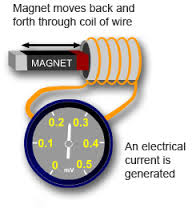Electromagnetic Induction was first discovered way back in the 1830’s by Michael Faraday. Faraday noticed that when he moved a permanent magnet in and out of a coil or a single loop of wire it induced an Electromotive Force or EMF. In other words a voltage, and therefore a current were produced. So what Michael Faraday discovered was a way of producing an electrical current in a circuit by using only the force of a magnetic field and not batteries. This then lead to a very important law linking electricity with magnetism, Faraday’s Law of Electromagnetic Induction.

The various topics that form a part of electromagnetic induction include

Faraday’s Laws of Electromagnetic Induction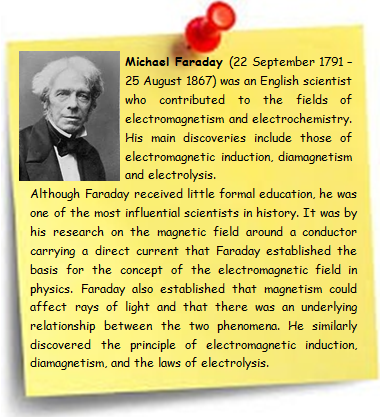?Lenz’s Law

Motion of a loop in a uniform magnetic field

Self-induction and co-efficient of self-induction

Mutual-induction

Co-efficient of mutual-induction

Energy stored in an inductor

Fleming’s right and left hand rule

Eddy current

Alternating current

Coil rotating in a uniform magnetic field

We shall give a brief outline of these topics in addition to the tips to master them. Those willing to go into the intricacies can refer the study material page on electromagnetic induction.

Important Formulas

Magnetic FluxInduced Electric Potential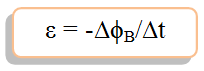Energy Stored in a Magnetic Field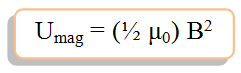Time Constant for an RL Circuit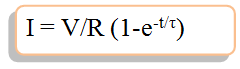Energy Stored in an Inductor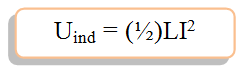Is this a valuable topic for those who are preparing for IIT-JEE, NEET (AIPMT) and CBSE Exam?

Electromagnetic induction is one of the easiest topics in physics, and one can easily score good marks in it. It is foolish to leave the questions from this section. Moderate levels of questions are coming from this section. The electromagnetic induction part contributes almost 5% in the IIT-JEE and the other two exams.

What are the best books for the preparation of electromagnetic induction?

Some of the books which are considered to be best for preparation of this section are:

Resnick Halliday

I.E. Irodov

H.C Verma

Problems of varied complexities can be found in the book Resnick Halliday. It has also a special edition dedicated to IIT-JEE. The standard of Irodov physics is quite high. At the end, H.C. Verma is quite useful for these examinations.

Illustrations

Question 1:- [IIT JEE 2014]

Two ideal batteries of emf V1 and V2 and three resistances R1, R2 and R3 areconnected as shown in the figure. The current in resistance R2 would be zero if

A.V1 = V2 and R1 = R2 = R3?            B.V1 = V2 and R1 = 2R2 = R3C.V1 = 2V2 and 2R1 = 2R2 = R3      D.2V1 = V2 and 2R1 = R2 = R3

Solution:-

Current through R2 will be zero of Eeqv of two batteries is zero

So, (V1/R1 – V2/R3)/(1/R1 + 1/R3) = 0 Or, V1/R1 = V2/R3

In option (A), V1 = V2 that gives, R1 = R3 whatever be the value

of R2 to maintain image of loop V1 with respect to V2.

Hence, option A and B both are correct.In options, C and D,

using loop analysis method we find,V1/V2 = 2, that satisfied, R3/R1 = 2

This relation is satisfied by option A &B.Hence, A, B and C are correct.

A point Q is moving in a circular orbit of radius R in the x-y plane with angular velocity ω. This can be considered as equivalent to a loop carrying a steady current Qω/2π. A uniform magnetic field along the positive z-axis is now switched on, which increases at a constant rate from 0 to B in one second. Assume that the radius of the orbit remains constant. The application of the magnetic field induces an emf in the orbit. The magnitude of the induced electric field in the orbit at any instant of time during the time interval of the magnetic field change, is

A.BR/4        B.BR/2        C.BR        D.2BR

Solution:-

E (2πR) = πR2 (dB/dt)
So, E = RB/2
So the option (B) is correct.Some Interesting Facts

Electromagnetic induction is the production of an electromotive (emf) in a conductor as a result of a changing magnetic field about the conductor.

The induced emf is always such that it opposes the change that gives rise to it, according to Lenz's law.

Changing the current in a given circuit can also induce an emf in another, nearby circuit unconnected with the original circuit; this type of electromagnetic induction, called mutual induction, is the basis of the transformer.

Electrostatic induction is the production of an unbalanced electric charge on an uncharged metallic body.

Magnetic induction is the production of a magnetic field in a piece of unmagnetized iron or other ferromagnetic substance when a magnet is brought near it.

Tips to Study Electromagnetic InductionElectromagnetic induction is one of the important chapter for the IIT-JEE aspirant’s. Often students doing mistake due to the lack of concept regarding the direction of electric- magnetic field and induced emf. Below are the some useful tips to handle this topic so quickly and accurately.

Always three parameters are involved in an electromagnetic induction problem. One is magnetic field, second one is a inductor or coil and the third one is movement of coil with respect to field.

Basically, in a given problem, two quantities are known and the third quantity needs to be calculated.

Before attempting the problem, check the units of all the parameters must belong to any one standard unit system. That is either in S.I or C.G.S.

Must aware of the Generator Rule which is the principle to determine the direction of induced emf of a conductor moving in a magnetic field.

Know the proper law and some concept regarding the direction of electric and magnetic field.

Clear your concept by doing the more and more problem on application of Faraday’s law, Lenz’s law, Fleming’s right hand and left hand thumb rule for current.

Since problems are directly coming from this section by using formulae and little concept, so try to save your time for difficult section like mechanicsPractical Applications of Electromagnetic Induction

Some electric generators can be driven by human power such as a hand crank or a motor car to generate electricity by the principle of electromagnetic induction.

A transformer uses two important connections between electricity and magnetism to convey power from one AC circuit to another results the application of electromagnetic induction.

An electric generator which a device for transforming mechanical energy to electrical energy to generate electricity is one of the applications of electromagnetic induction.

The bulb in the halogen desk lamp operates from low voltages provided with the help of a transformer, which based on the theory of electromagnetic induction.

A hybrid automobile couples together a combustion engine and one or more motor/generators so that it can convert energy easily between mechanical and electric forms and can operate on fuel, battery, or both by the help of electromagnetic induction principle.

Loudspeakers use electromagnets to vibrate the cone and produce sound based on the principle electromagnetic induction.

Using electromagnetic induction, powerful magnets can be turned on and off using electricity, unlike permanent magnets.

Radio’s, televisions, refrigerators are all contains transformers resulting uses of electromagnetic induction principle.

The ignition system of a car as well as starter are based on the theory of electromagnetic induction

In a commercial electric power plant, large generators produce energy that is transferred out of the plant by electric transmission. These generators use magnetic induction to generate a potential difference when coils of wire in the generator are rotated in a magnetic field.

```### Course Features

• 101 Video Lectures
• Revision Notes
• Previous Year Papers
• Mind Map
• Study Planner
• NCERT Solutions
• Discussion Forum
• Test paper with Video Solution